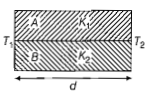• Subject:

...

• Topic:

...

Two rods A and B of different materials are welded together as shown in figure. Their thermal conductivities are ${k}_{1}$ and ${k}_{2}$. The thermal conductivity of the composite rod will be(a) $\frac{{K}_{1}+{K}_{2}}{2}$

(b) $\frac{3\left({K}_{1}+{K}_{2}\right)}{2}$

(c) ${K}_{1}+{K}_{2}$

(d) $2\left({K}_{1}+{K}_{2}\right)$

NEET